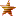###Author Topic: Quadratic Formula  (Read 52 times)

#### PiotrGrochowski

• Administrator
• Newbie
•• Posts: 4##### Quadratic Formula
« on: September 19, 2020, 04:46:52 pm »
The main way to turn quadratic outlines into pixel borders. This must be done twice horizontally and once vertically for correct rendering, for proper handling of half pixel corners later. This step is followed by Pixel Building inside the borders, done in one pass each for the top and bottom edges, and two passes each for left and right edges. The possibility of missing extrema is ignored.

First, we have to turn the Quadratic Bezier into a Quadratic Polynomial: for each dimension, given the points a, b, c, the quadratic function where f(0)=a and f(1)=c (curve goes from 0 to 1) is (a)x⁰+(2*(b-a))x¹+((c+a)-(2*b))x².

We have the quadratic polynomial, (q)x⁰+(w)x¹+(e)x². In both horizontal and vertical dimensions, where the curve goes from 0 to 1.

We apply this rule: For each half pixel position (let's say, j) along a dimension we place an edge. First we apply the Quadratic Formula to determine at what location on the curve the coordinate is located.
It is located at
double r = j-q; double t = w/(-2.0*e); double y; if(1.0/t == 0.0) y = r/w; else if(t<0.5) y = t+sqrt((t*t)+(r/e)); else y = t-sqrt((t*t)+(r/e)); return y;
With the location now known, it is possible to determine where the edge would be. On the other dimension, we simply do (q)x⁰+(w)x¹+(e)x² with the obtained location. It must then be rounded to nearest integer. For half-rounding note that because pixels on TOP of the outline are inside, half-edges on the top and right are rounded up, while half-edges on the bottom and left are rounded down.
The Quadratic Formula is absolutely necessary to rasterize quadratic outlines. It can be figured out with a lot of mathematical thinking, however it is very common to make mistakes in implementation of it.

#### PiotrGrochowski

• Administrator
• Newbie
•• Posts: 4##### Re: Quadratic Formula
« Reply #1 on: September 26, 2020, 06:27:42 pm »
The best known way is Six Pass Pixel Building:

1. Trace all edges on the left side, rounding the vertical boundaries of bezier curve to the nearest (if half, up) and increment the pixel values of all pixels to the right. (first pass)
2. Trace all edges on the right side, rounding the vertical boundaries of bezier curve to the nearest (if half, up) and decrement the pixel values of all pixels to the right. (second pass)
3. Create a new pixel buffer, and do steps 1 and 2 but rounding half down instead. (third and fourth pass)
4. Merge the pixel buffer into the main buffer by taking the maximum value of each.
5. Trace all edges on the top side, rounding the horizontal boundaries of bezier curve to the nearest (if half, up) and increment the pixel values of all pixels below. (fifth pass)
6. Trace all edges on the bottom side, rounding the horizontal boundaries of bezier curve to the nearest (if half, up) and decrement the pixel values of all pixels below. (sixth pass)
7. Now each inside pixel is of value 2, or 1. Each value greater than 0 or less than -1 is a filled pixel.

Done! Now go do dropout control on https://pixelbuilding.createaforum.com/quadratic-pixel-building/dropout-control/